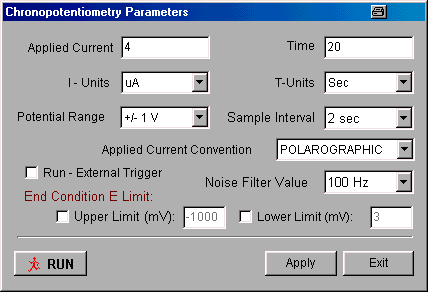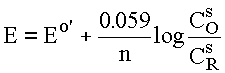# Chronopotentiometry

#### Introduction

Epsilon instruments contain both a potentiostat and a galvanostat, and hence can perform both controlled potential (potentiostatic) and controlled current (galvanostatic) experiments. Although potentiostatic experiments are much more common, there are some applications for which a galvanostat is advantageous.

The galvanostat uses a three electrode configuration, in which a current is applied between the auxiliary and working electrodes, and the potential of the working electrode (measured with respect to the reference electrode) is monitored. The basis of controlled current experiments is that a redox (electron transfer) reaction must occur at the surface of the working electrode in order to support the applied current. For example, if ferricyanide is present in the solution, then a reducing current will lead to the reduction of ferricyanide to ferrocyanide at the working electrode (note that a balancing oxidation must also occur at the auxiliary electrode). Common applications of the galvanostat include constant current stripping potentiometry and constant current electrolysis (including applications where a constant rate of electrolysis in important, such as electrodeposition and battery studies). One advantage of all constant current techniques is that the ohmic drop due to solution resistance is also constant, as it is equal to the product of the current and the solution resistance. The ohmic distortion can therefore be simply corrected by a constant potential offset. In contrast, in potentiostatic experiments (e.g., cyclic voltammetry), the current, and hence the ohmic drop, varies with potential, and correction is more complicated.Figure 1. Current excitation signal for a chronopotentiometry experiment.

Chronopotentiometry (CP) is the most basic constant current experiment and is a standard technique for the epsilon. In CP, a current step is applied (Fig1) across an electrochemical cell (without stirring). In double step chronopotentiometry (DSCP), a second current step is applied (Fig2). It should be noted that the time scale of a DSCP experiment is typically shorter (seconds or milliseconds) than that of CP experiment (minutes or seconds). The protocol for defining the sampling rate is therefore different for the two techniques. CP is a standard technique, whereas DSCP is only available as an optional addition.Figure 2. Current excitation signal for a double step chronopotentiometry experiment.

#### Setting Up a Chronopotentiometry Experiment

The parameters required for a CP experiment are the current value and the length of the current step. The values of these parameters are entered using the Change Parameters dialog box (Fig3) in either the Experiment menu or the pop-up menu.Figure 3. Change Parameters dialog box for chronopotentiometry.

1. Current values are entered in mA, mA, nA, or pA (selected using I - Units), and time values are entered in minutes or seconds (selected using T-Units).
2. If the Run - External Trigger box is checked, the experiment is started from an external device using the Start In back-panel connection.
3. The rate of data acquisition is determined by the Sample Interval (e.g., the default rate is one point every second).
4. The current polarity is determined by the Applied Current Convention - IUPAC = positive oxidation current, Polarographic = positive reduction current
5. The Noise Filter Value determines the amount of analog filtering.
6. The end of the experiment can determined by the Time or by the End Condition E Limit in mV (if the appropriate check box is checked). The experiment can also be ended manually.
7. Clicking Exit will exit the dialog box without saving any changes made to the parameter values. Any changes can be saved by clicking Apply before exiting.
8. The Multi-Run option is not available for this technique.
9. Range of allowed parameter values:
• Current = 50 pA - 50 mA (but note that there are accuracy limitations when applying sub-nA currents)
• End Condition E Limit = -999 - +999 mV (for Potential Range = +/- 1V), or -9999 - +9999 mV (for Potential Range = +/- 10V)
• Sample Interval = 0.05, 0.1, 0.2, 0.5, 1, 2, 5, 10, 30, 60 sec
• The largest number that can be entered for Time is 32,000. However, the maximum number of data points that can be collected in one experiment is 64,000, so the maximum value for the Time of a given experiment is also determined by the Sample Interval.
10. Once the parameters have been set, the experiment can be started by clicking Run (either in this dialog box, in the Experiment menu, in the pop-up menu, on the Tool Bar, or using the F5 key).

#### Setting Up a Double Step Chronopotentiometry Experiment

As shown in Fig4, four parameters are used in the epsilon software to define the current wave form for DSCP.

1. First Step Current
2. Second Step Current
3. First Step Time
4. Second Step Time

The values of these parameters are entered using the Change Parameters dialog box (Fig4) in either the Experiment menu or the pop-up menu.Figure 4. Change Parameters dialog box for double step chronopotentiometry.

1. Current values are entered in mA, mA, nA, or pA (selected using Current Units), and time values are entered in seconds or milliseconds (selected using T-Units).
2. If the Run - External Trigger box is checked, the experiment is started from an external device using the Start In back-panel connection.
3. The rate of data acquisition is determined by the Sample Interval (e.g., the default rate is one point every second).
4. The current polarity is determined by the Applied Current Convention - IUPAC = positive oxidation current, Polarographic = positive reduction current
5. The Noise Filter Value determines the amount of analog filtering. The correct filter will be set automatically if Auto Filter is checked.
6. Clicking Exit will exit the dialog box without saving any changes made to the parameter values. Any changes can be saved by clicking Apply before exiting.
7. The Multi-Run option is not available for this technique.
8. Range of allowed parameter values:
• Step Current = 50 pA - 32 mA (but note that there are accuracy limitations when applying sub-nA currents)
• Step Time = 1 - 65 seconds OR 1 - 16000 milliseconds
• Maximum # of points in a step = 1000, 2000, 4000, 8000, 16000
• The Sample Interval is determined by the Step Time and the Maximum # of points in a step, and can only be adjusted by the user indirectly through these latter two parameters. The relationship between these parameters is shown by the equation

Sample Interval = Step Time/Maximum # of points

However, it should be noted that only certain values are allowed for each of these parameters, as is shown in the table below:

 Max. # of points 1000 2000 4000 8000 16000 Sample Interval Maximum Step Time (/ms) 20 ms 20 40 80 160 320 50 ms 40 81 162 325 650 100 ms 100 200 400 800 1600 200 ms 200 400 800 1600 3200 500 ms 406 812 1625 3250 6500 1 ms 1000 2000 4000 8000 2 ms 2000 4000 8000 5 ms 4062 8125 10 ms 10000

9. Once the parameters have been set, the experiment can be started by clicking Run (either in this dialog box, in the Experiment menu, in the pop-up menu, on the Tool Bar, or using the F5 key).

#### Analysis of the Potential vs. Time Curve

The shape of the potential response can be rationalized by considering the concentration profiles of the redox species as a function of time (Fig5).Figure 5. Concentration profiles for chronopotentiometry.

Let us consider the electron transfer reaction O + e- = R. Before the current step, the concentration of O at the electrode surface is the same as in the bulk solution (i.e., 5 mM). The initial potential is the rest potential or the open circuit potential (Eo.c.). Once the (reducing) current step has been applied, O is reduced to R at the electrode surface in order to support the applied current, and the concentration of O at the electrode surface therefore decreases. This sets up a concentration gradient for O between the bulk solution and the electrode surface, and molecules of O diffuse down this concentration gradient to the electrode surface. The potential is close to the redox potential for O + e- = R, and its precise value depends upon the Nernst equation:where CsO and CsR are the surface concentrations of O and R, respectively. These concentrations vary with time, so the potential also varies with time, which is reflected in the finite slope of the potential vs. time plot at this stage. Once the concentration of O at the electrode surface is zero, the applied current can no longer be supported by this electron transfer reaction, so the potential changes to the redox potential of another electron transfer reaction. If no other analyte has been added to the solution, the second electron transfer reaction will involve reduction of the electrolyte; that is, there is a large change in the potential (Fig6).Figure 6. Typical potential response for a chronopotentiometry experiment.